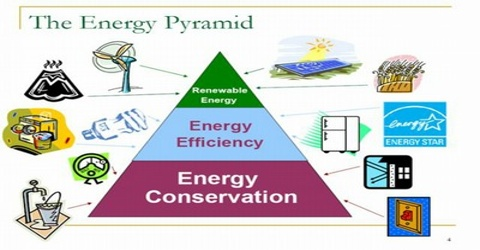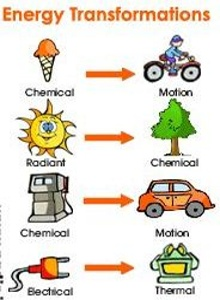Physics

# Application of the Principle of Conservation of EnergyApplication of the Principle of Conservation of Energy

When two palms are rubbed together, they become warm; here mechanical energy is converted into heat energy. When a falling body strikes the ground and get stopped, the mechanical energy is converted into heat energy and some sound energy. Again, due to friction between different parts of a machine heat energy is created. From the above events, it is observed that energy is converted from one form to another form. Further, it is seen from Einstein’s theory of relativity that mass is converted into energy. If energy of a body increases, its mass also increases. On the other hand, if energy decreases in a body, its mass also decreases. When a box is pulled over the floor heat is produced due to friction.

It is seen from all the above cases (conservative or non-conservative) that energy is converted from one form to another form, but is never exhausted or destroyed. This is the principle of conservation of energy.

Law: “Energy can neither be created nor destroyed but can only be convened from one form to another.”

The total energy of the universe is Constant. In electric iron, heat is produced when electricity is passed through it. We iron our clothes with this heat. In this case, electric energy is convened to heat energy and finally heat energy is converted to mechanical energy. Here no energy is destroyed, only there is transformation.You have heard about nuclear reactor. In nuclear reactor nuclear fission is created by bombarding a heavy nucleus (235U92) by a neutron. In this reaction enormous amount of heat energy is produced. Electricity is produced by rotating turbines by using this heat energy. In this case it is seen that nuclear energy is converted into heat energy and heat energy is converted into electric energy. In this case also there is no loss or destruction of energy. Only energy is transformed from one form to another form.

When energy is changed from one form to another form, then no increase or decrease of energy occurs. That means, either creation or destruction of energy is impossible. When one form of energy destroyed, it appears in another form. It is called the conservation principle of energy.